# Inter Maths Solutions for Applications of Derivatives exercise 10(d)

Intermediate Mathematices 1B Solutions for exercise 10(d) Applications of Derivatives are given.

Study the textbook lesson Applications of Derivatives very well.

Practice the example problems and solutions given in the textbook.

Observe the solutions given below and try them in your own method.

You can also see

Inter Maths 1A textbook solutions

Inter Maths 1B textbook solutions

Inter Maths IIA textbook solutions

Inter Maths IIB textbook solutions

Applications of Derivatives

Exercise 10(a)

Exercise 10(b)

Exercise 10(c)

Exercise 10(d)

Exercise 10(e)

Exercise 10(f)

Exercise 10(g)

Exercise 10(h)

M

## Maths Intermediate Solutions for Applications of Derivatives exercise 10(d)## Exercise 10(d) solutions Inter maths 1B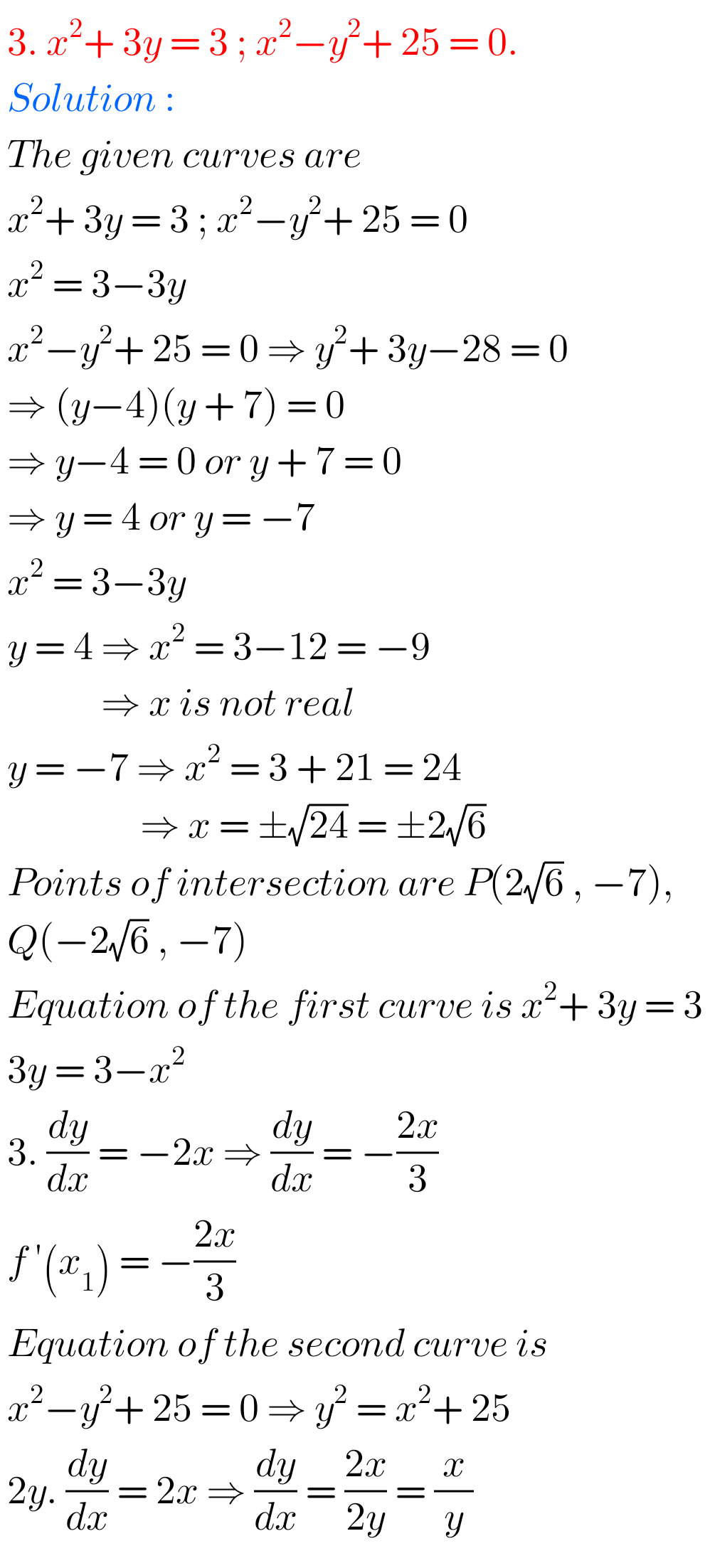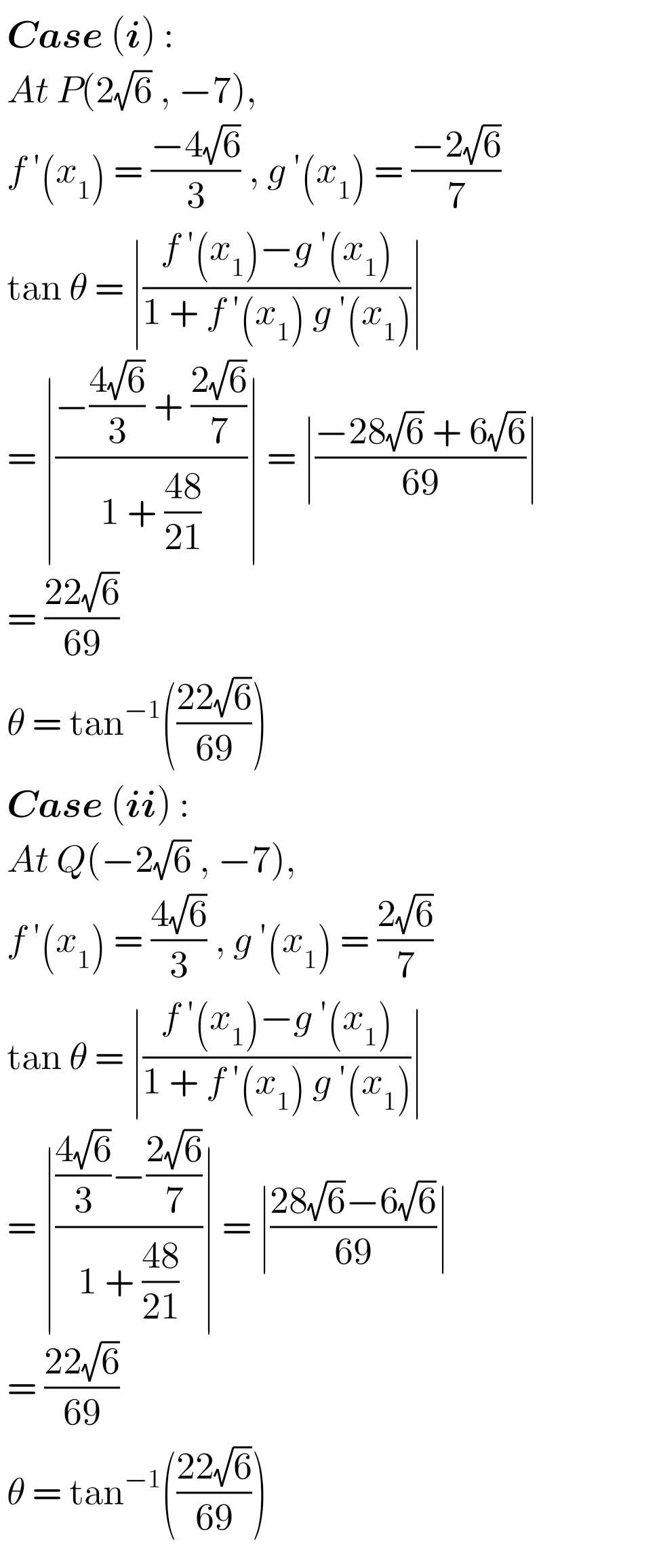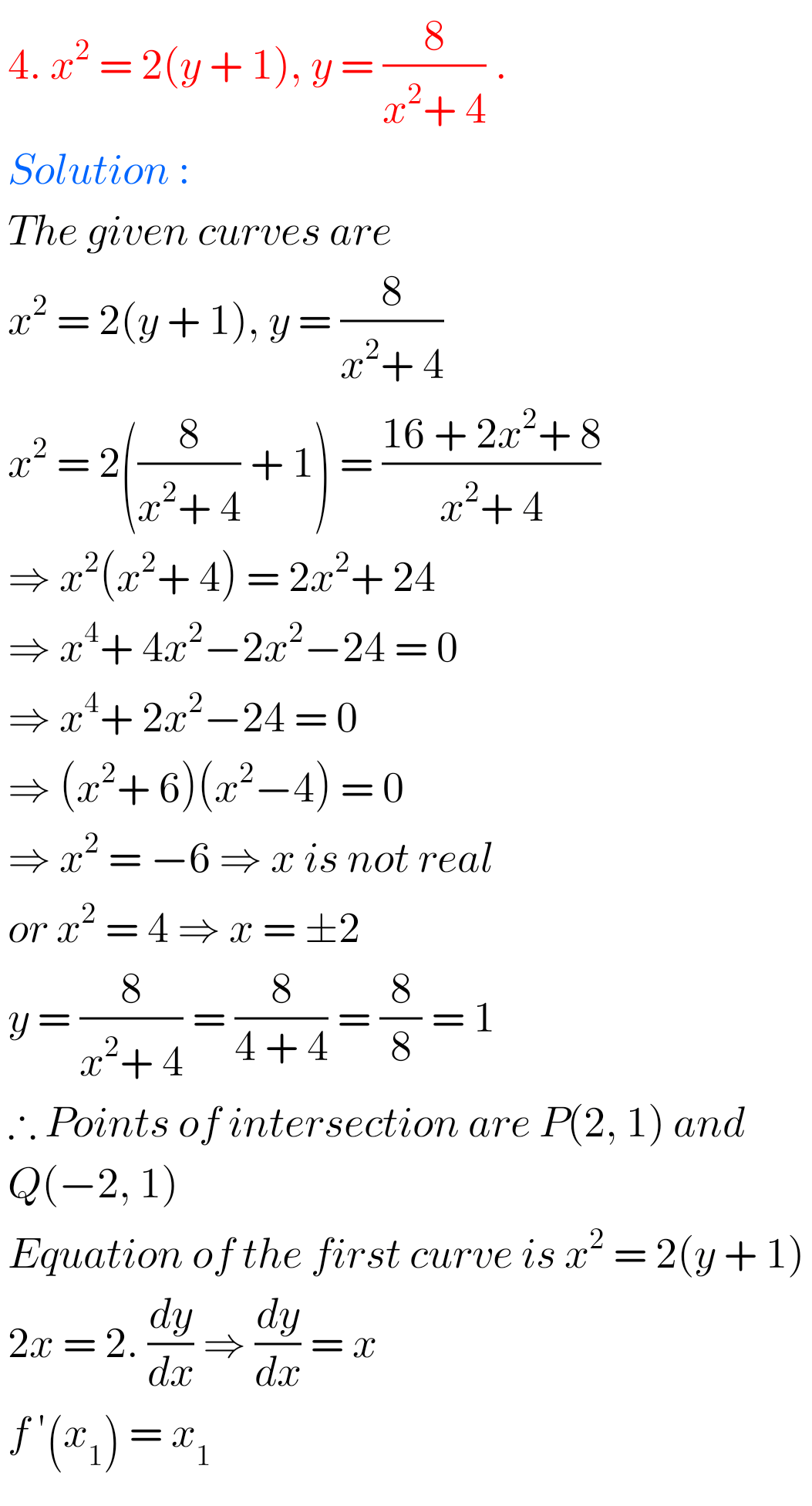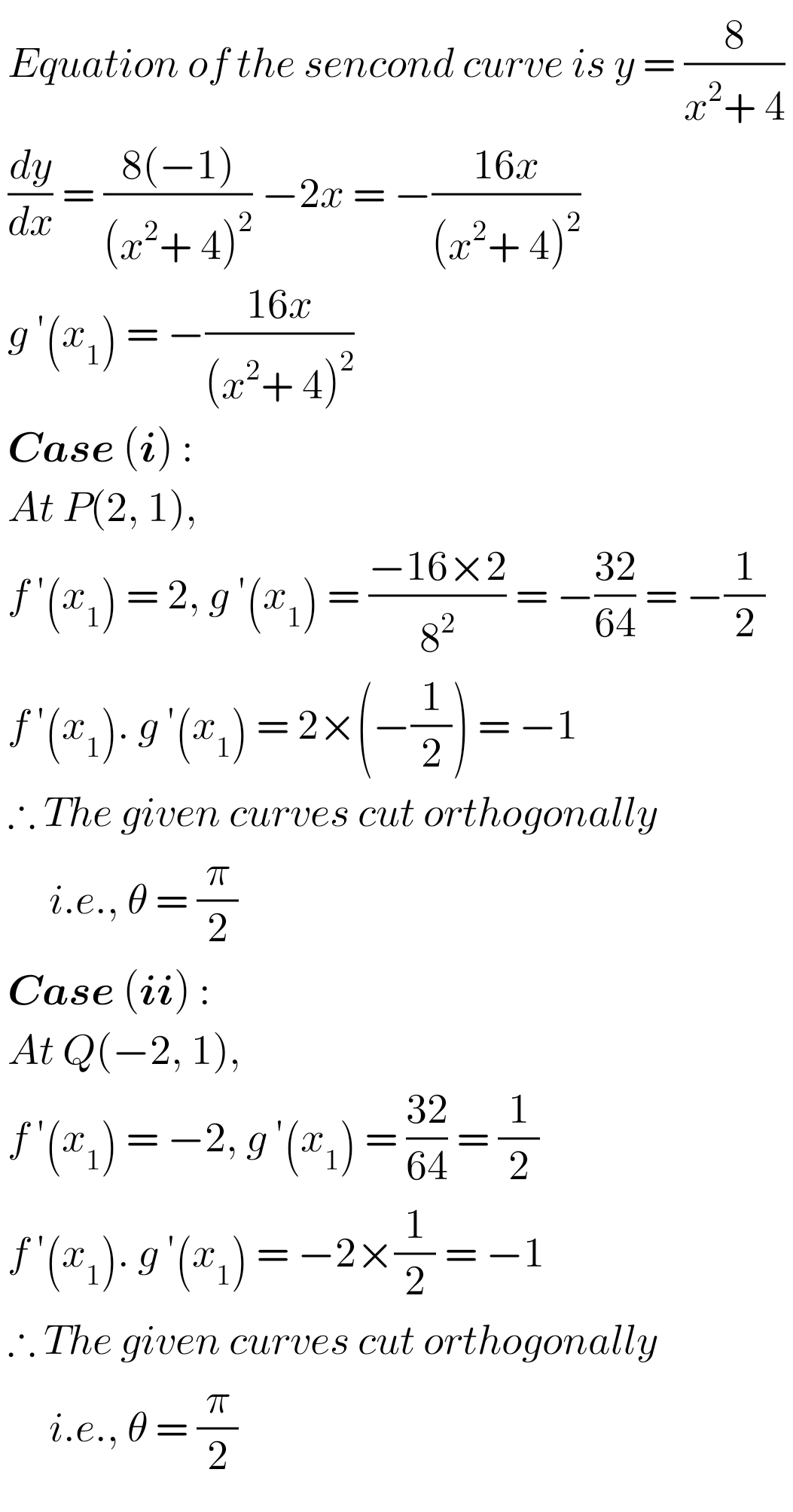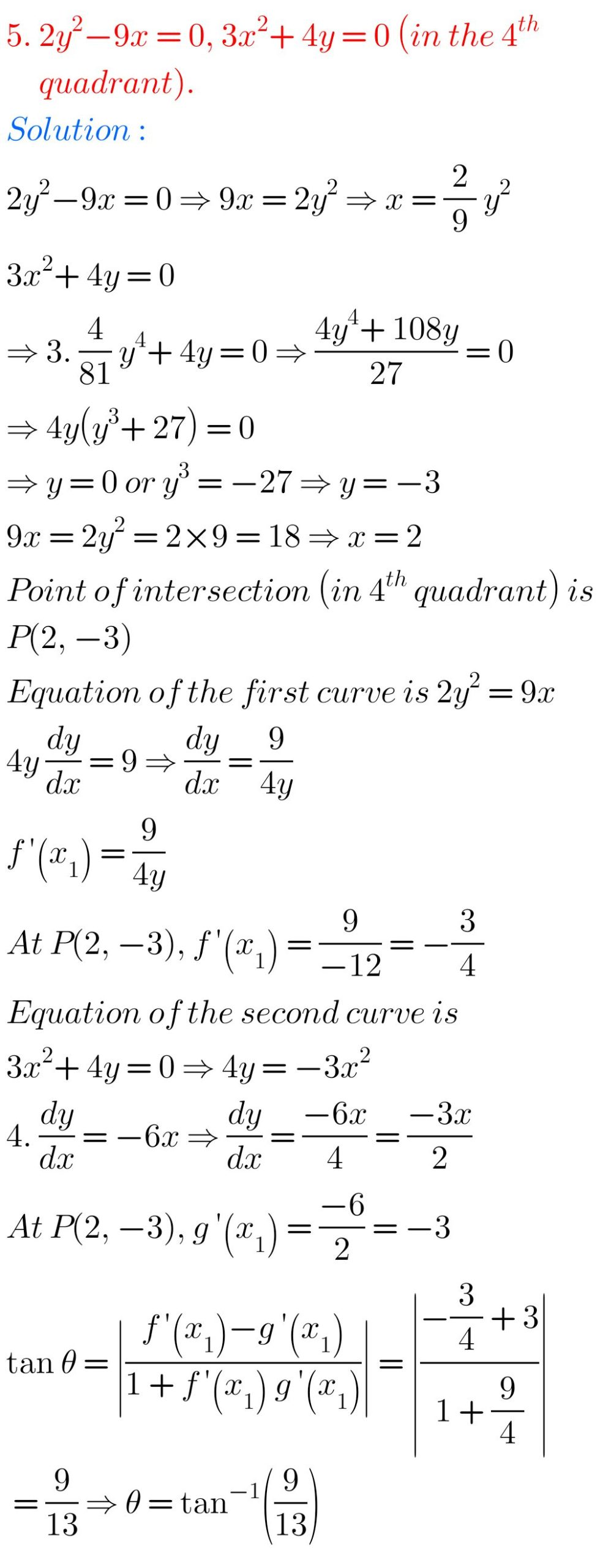## Chapter 10 exercise 10(d) solutions Inter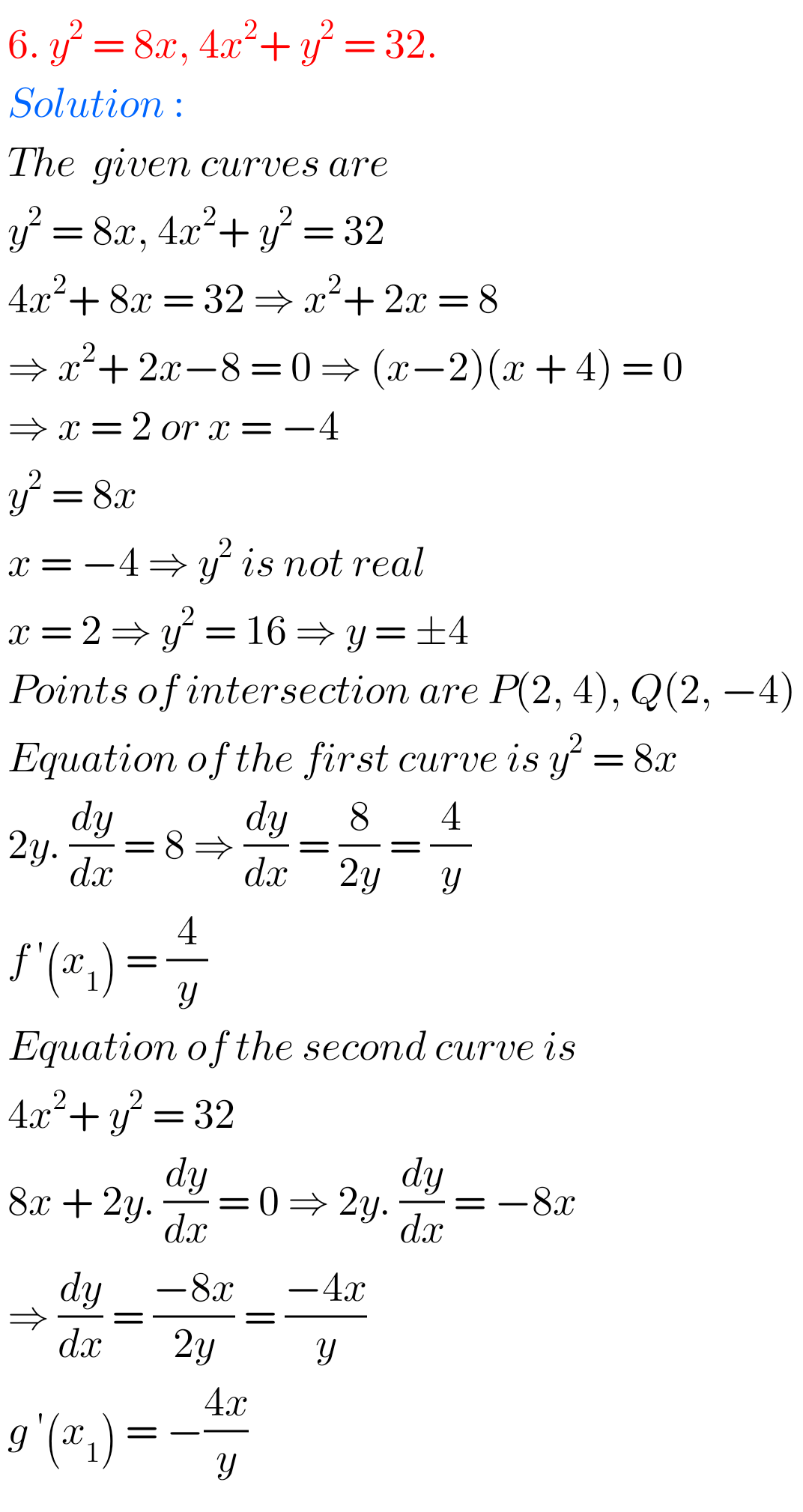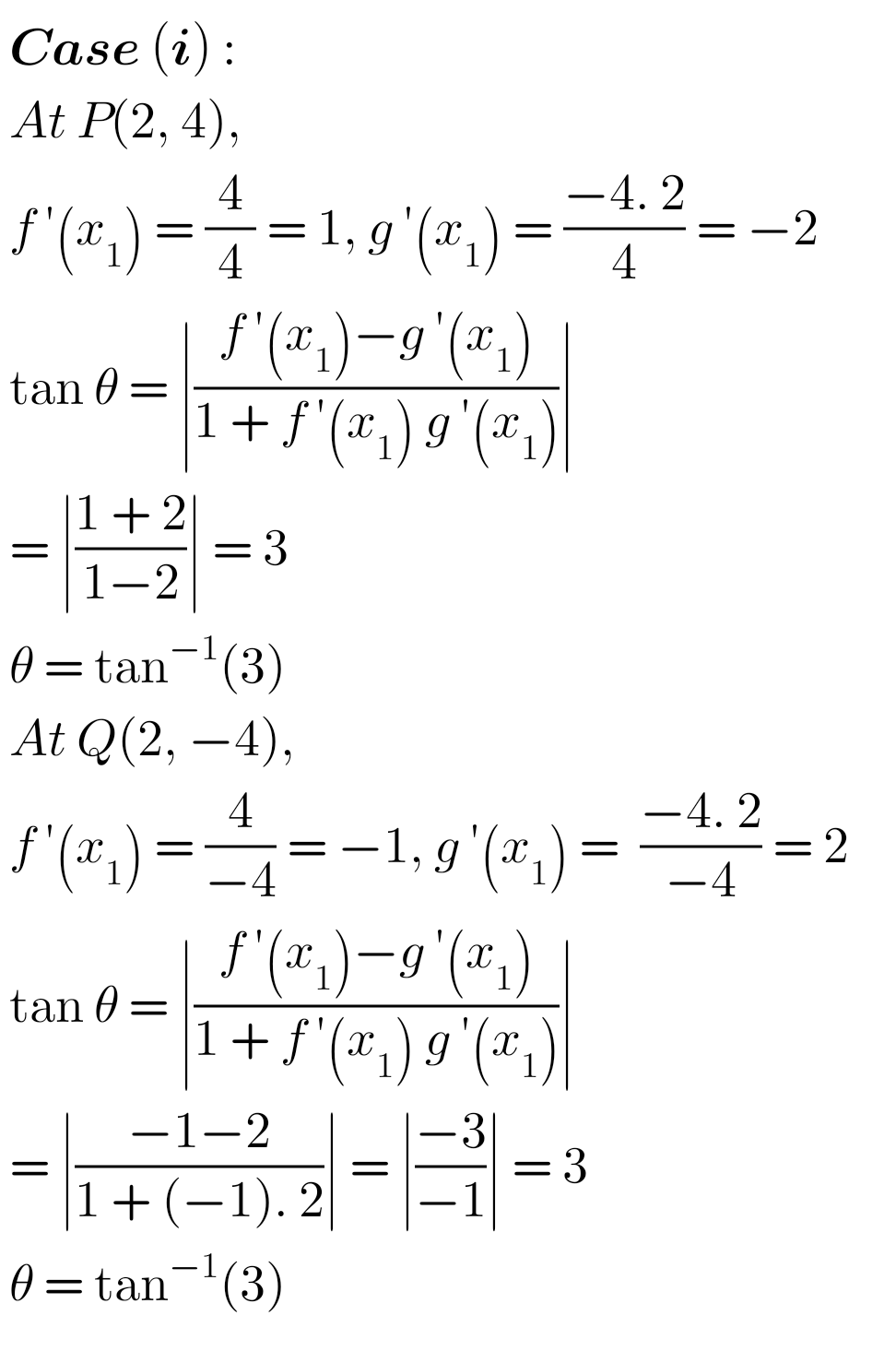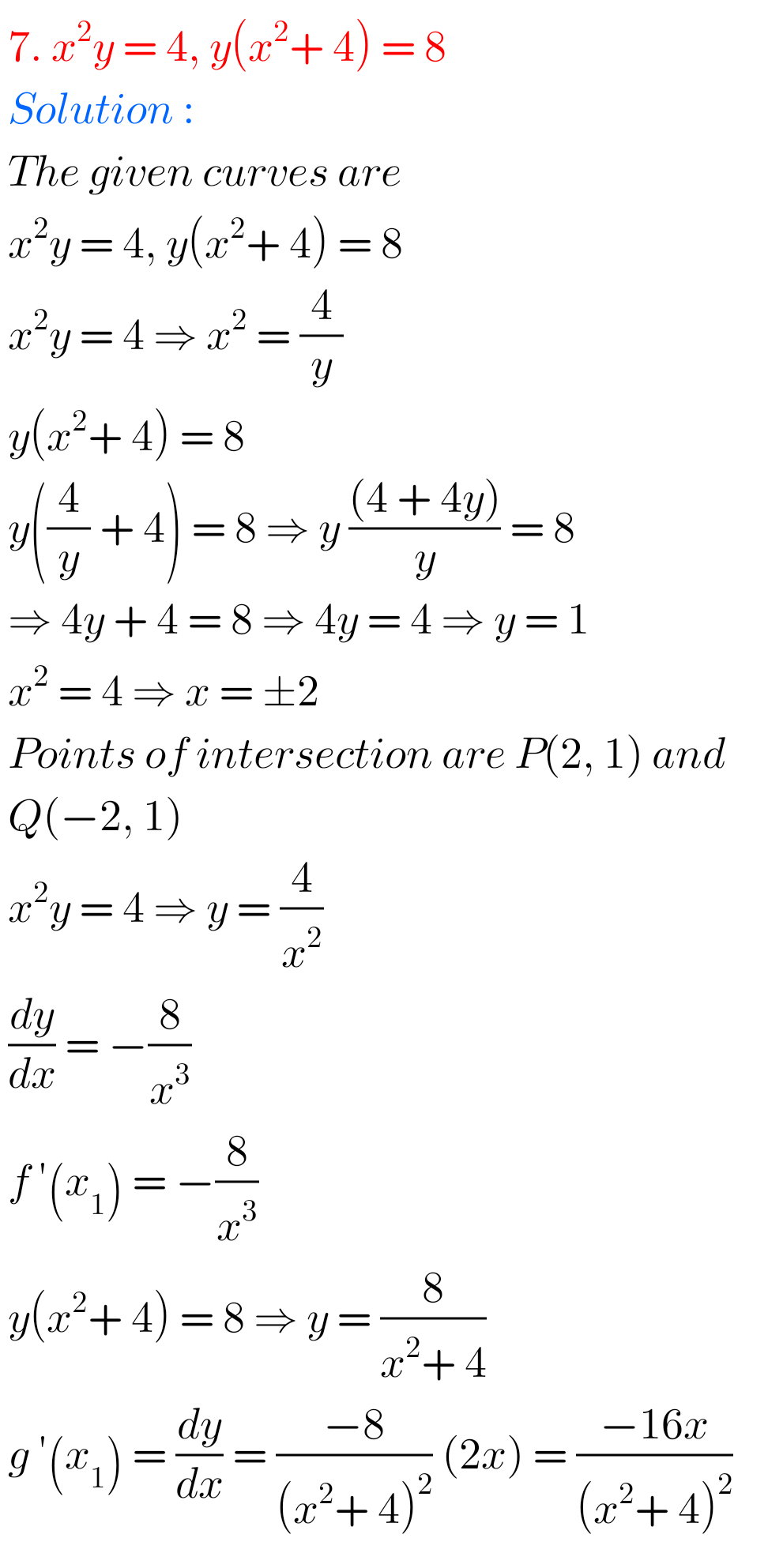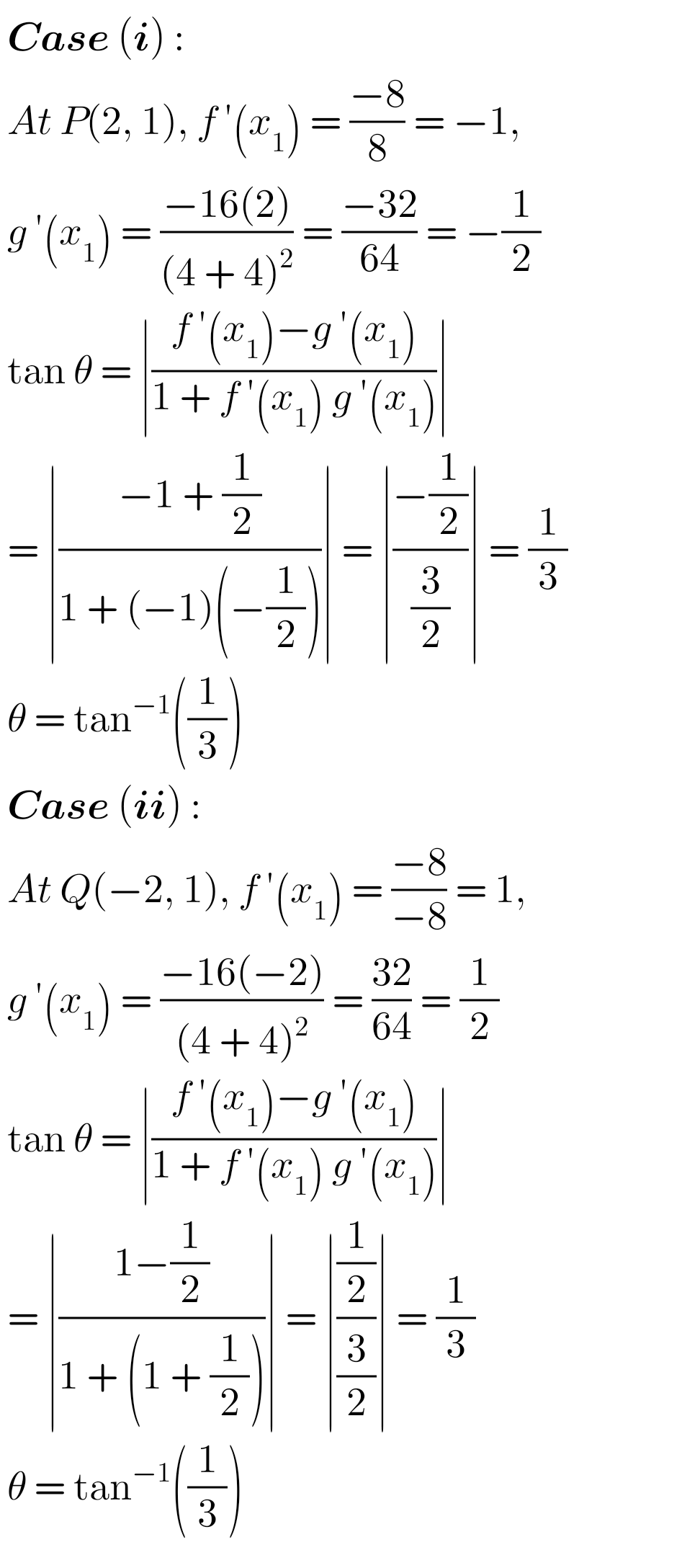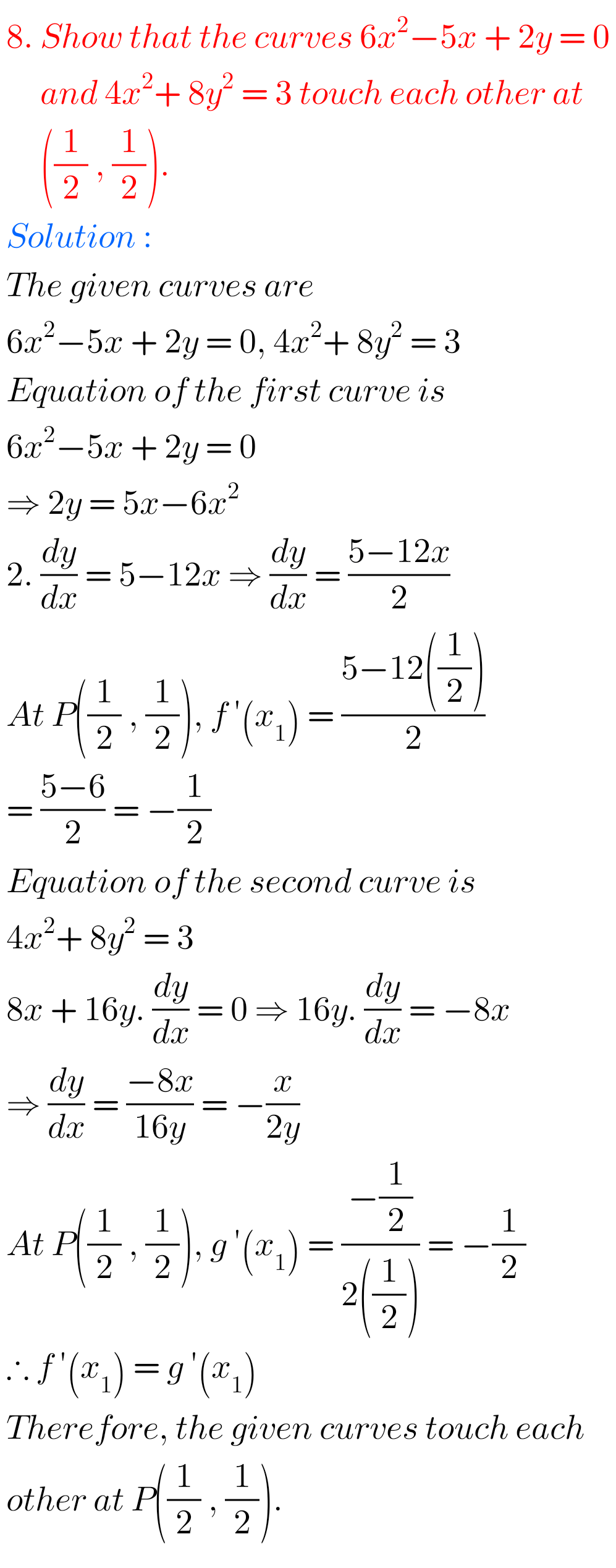Note : Observe the solutions and try them in your own method.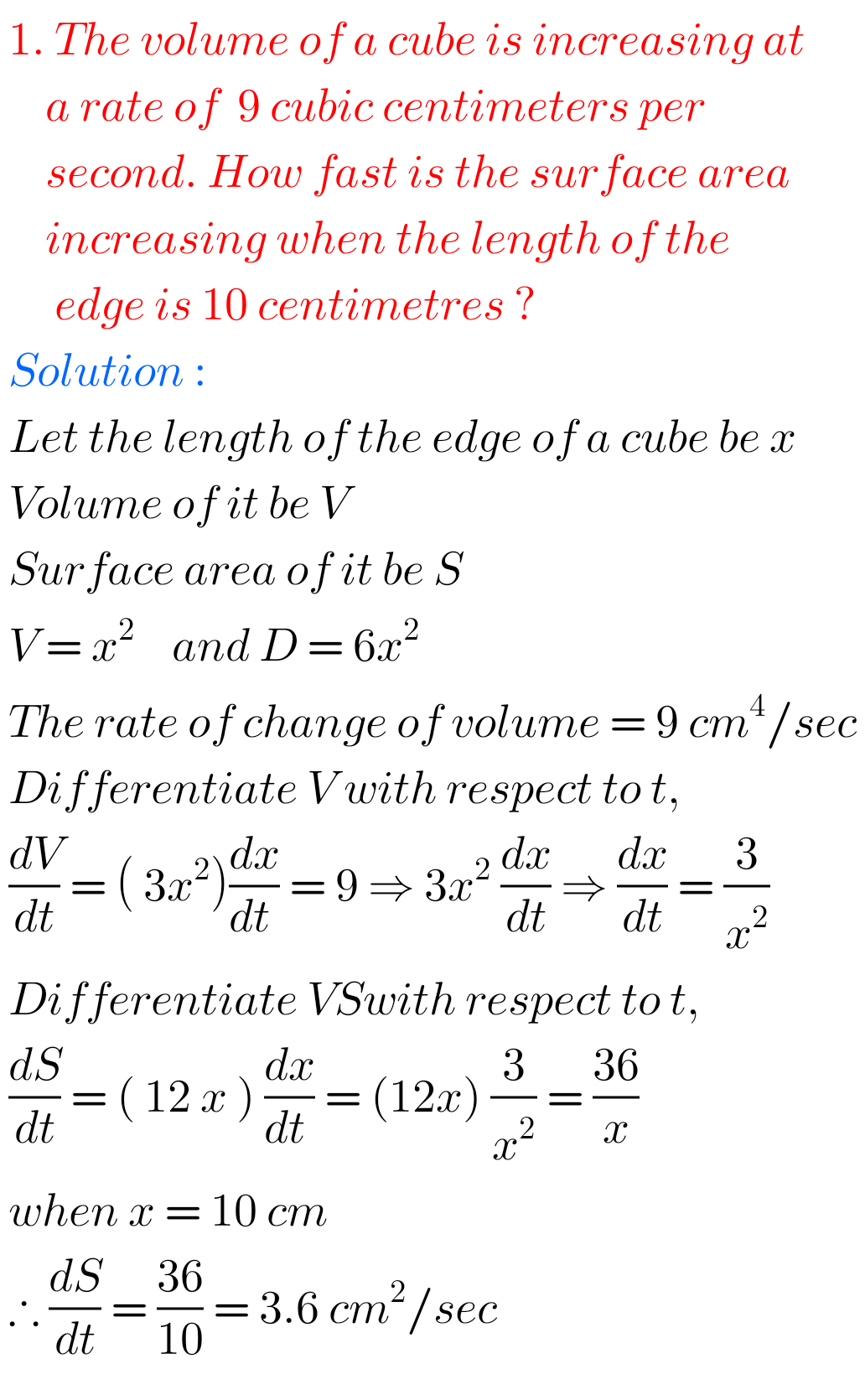SSC Maths Solutions class 10